# Blackboard Shots with Prefix "Abbasi"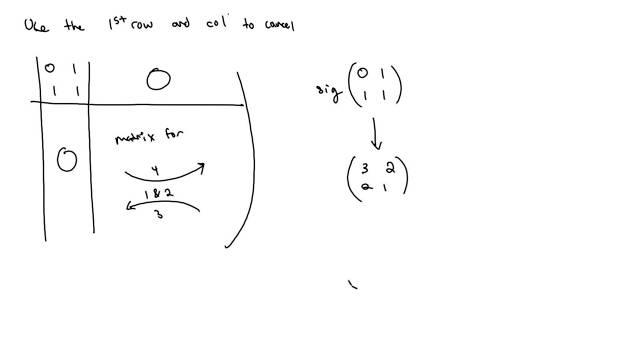211109-145132: Proof of invariance of Kashaev's signature (6).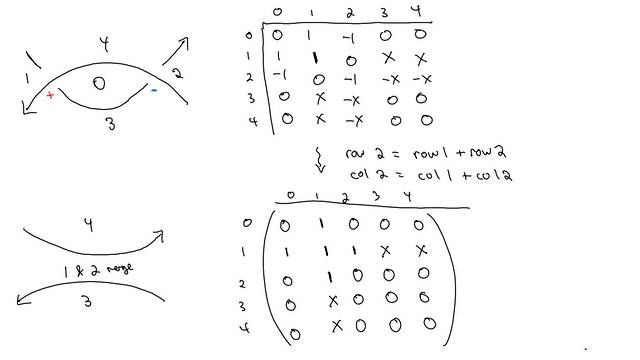211109-145131: Proof of invariance of Kashaev's signature (5).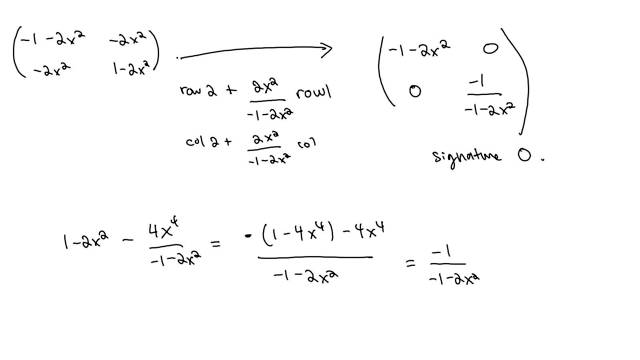211109-145130: Proof of invariance of Kashaev's signature (4).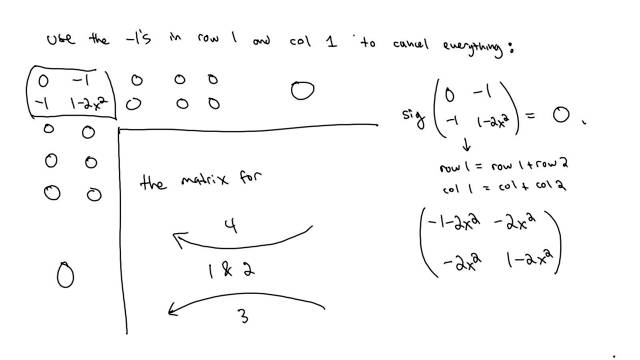211109-145129: Proof of invariance of Kashaev's signature (3).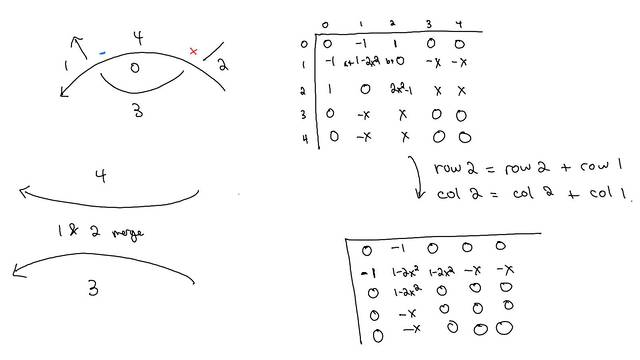211109-145128: Proof of invariance of Kashaev's signature (2).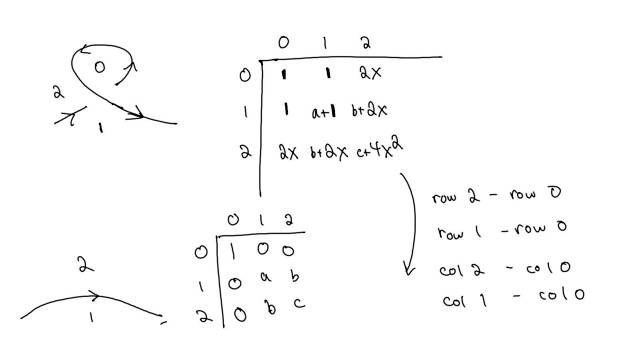211109-145127: Proof of invariance of Kashaev's signature.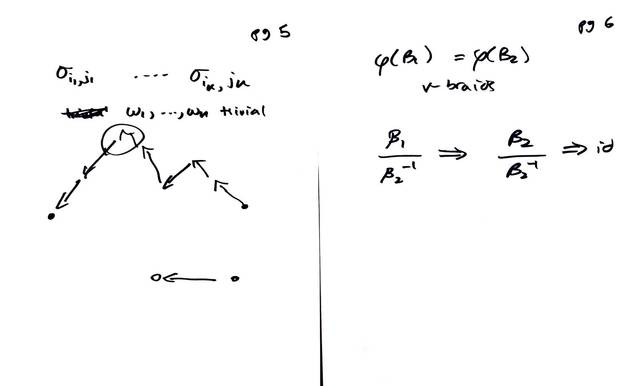200717-173027: A short proof of McCool's theorem (3).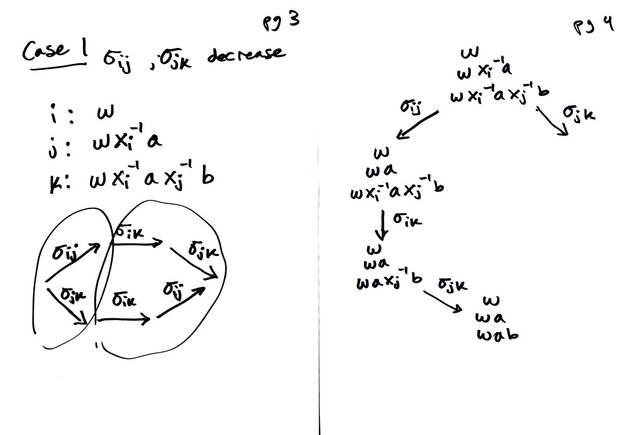200717-173026: A short proof of McCool's theorem (2).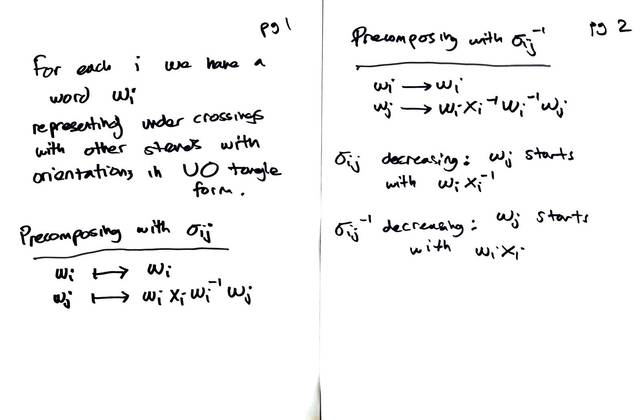200717-173025: A short proof of McCool's theorem.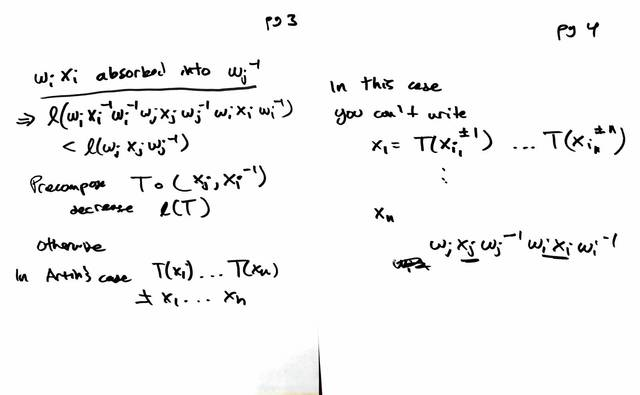200630-181150: Basis conjugating automorphisms are generated by $\sigma_{ij}$ (2).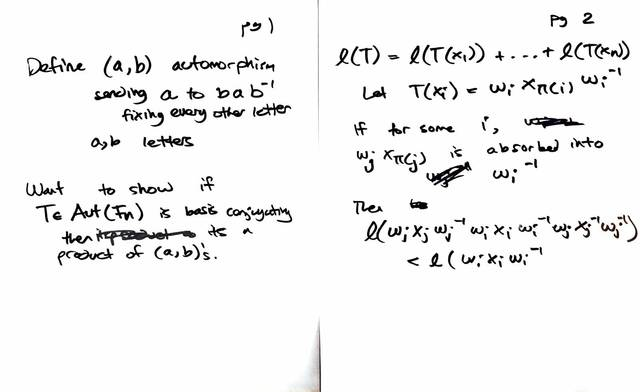200630-181149: Basis conjugating automorphisms are generated by $\sigma_{ij}$.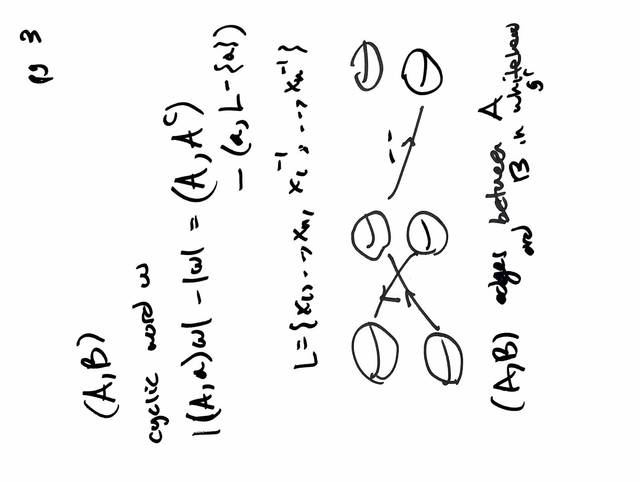200627-114335: Wormholes on $S^3$ (2).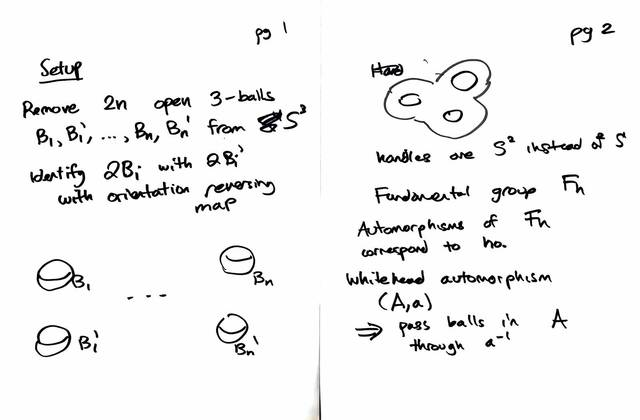200627-114334: Wormholes on $S^3$.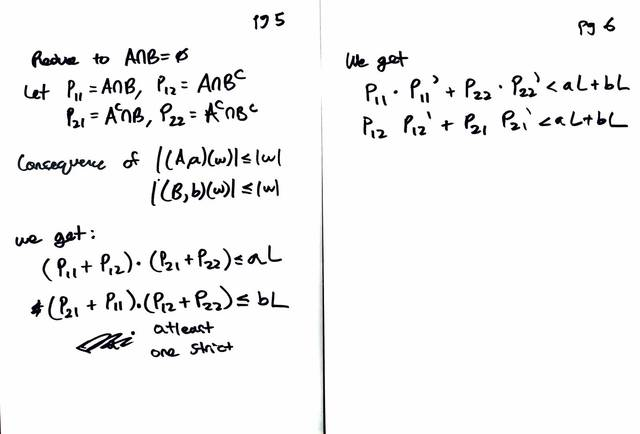200619-160001: Towards a proof of McCool's theorem (7).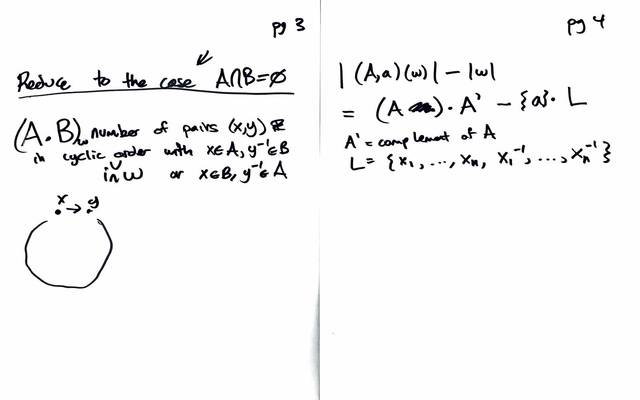200619-160000: Towards a proof of McCool's theorem (6).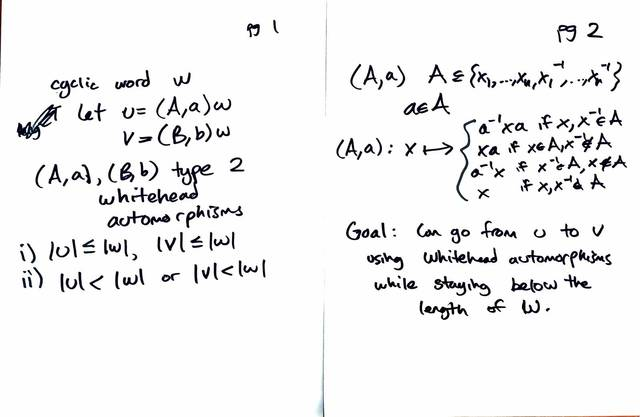200619-155959: Towards a proof of McCool's theorem (5).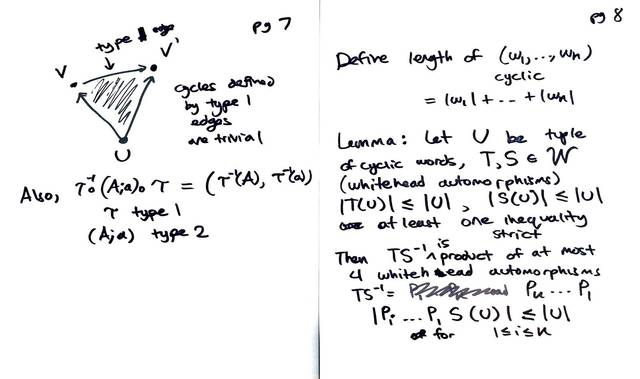200616-182321: Towards a proof of McCool's theorem (4).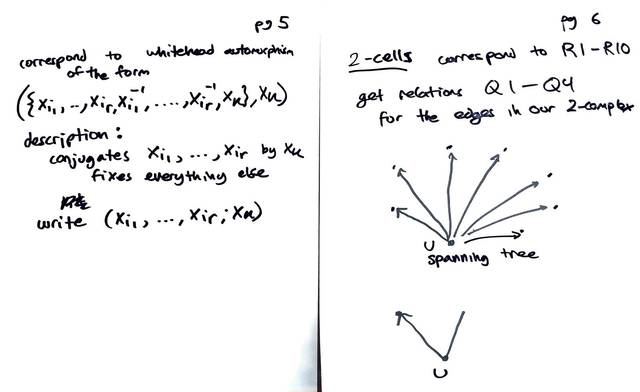200616-182320: Towards a proof of McCool's theorem (3).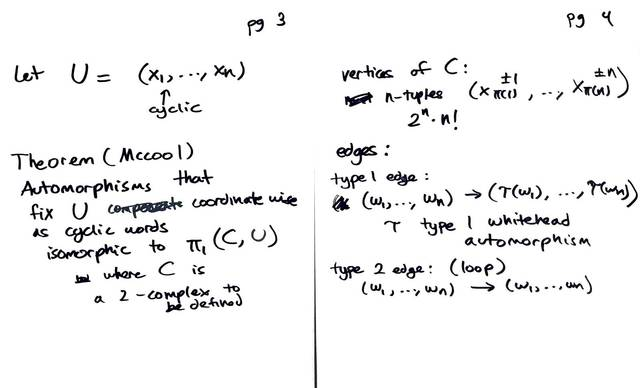200616-182319: Towards a proof of McCool's theorem (2).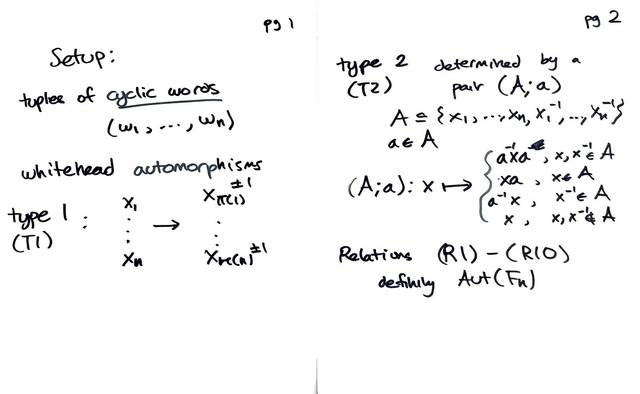200616-182318: Towards a proof of McCool's theorem.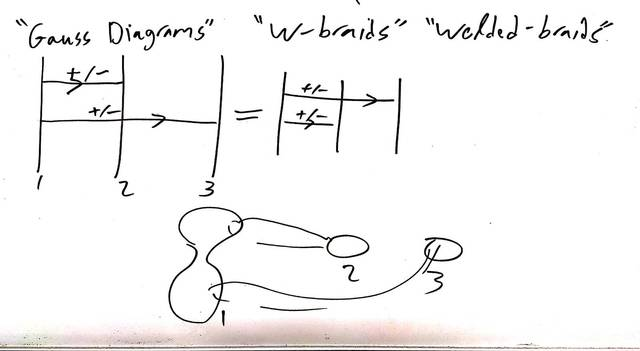200611-161506: Gauss diagrams, w-braids.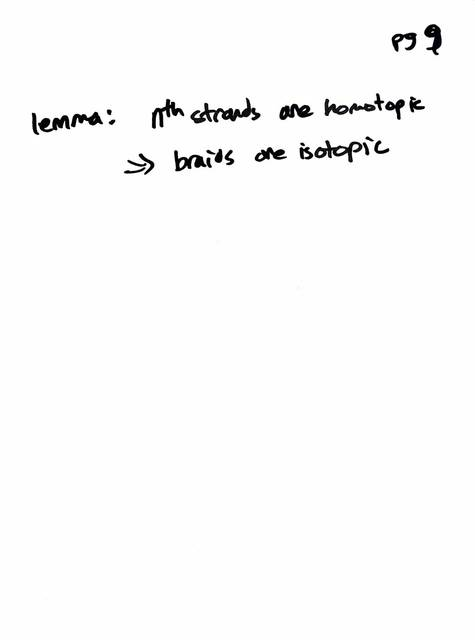200529-165625: Proof of injectivity (5).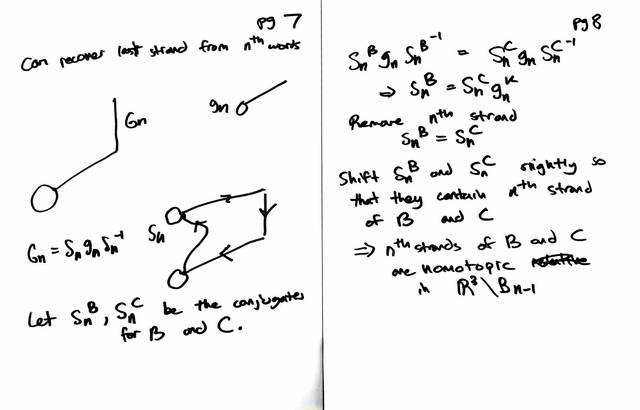200529-165624: Proof of injectivity (4).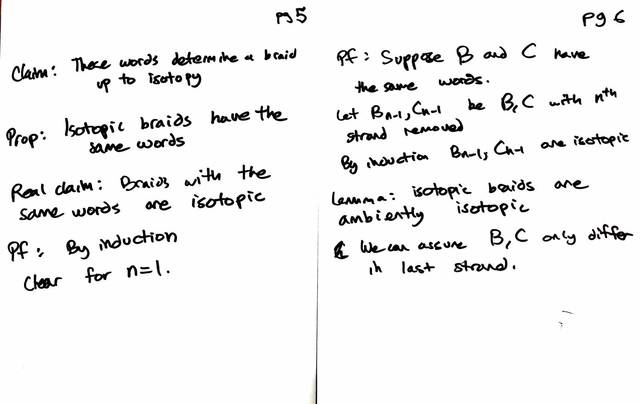200529-165623: Proof of injectivity (3).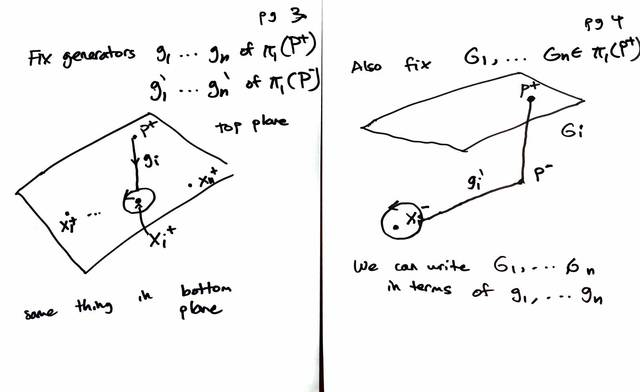200529-165622: Proof of injectivity (2).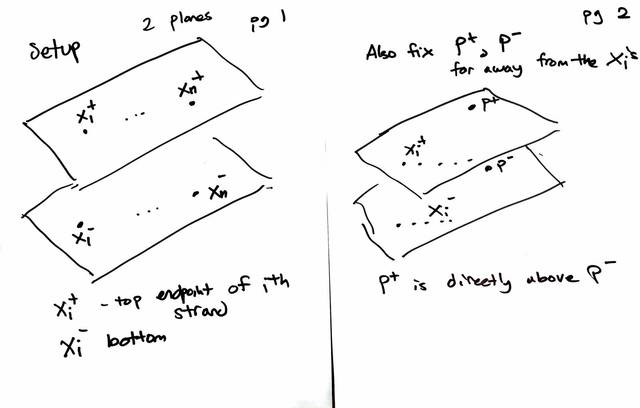200529-165621: Proof of injectivity.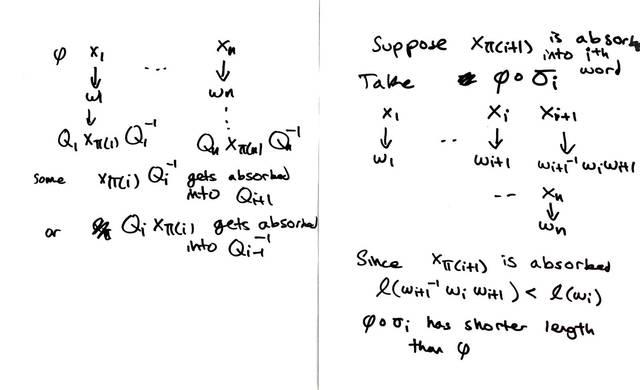200515-165502: Proving Artin's theorem (2).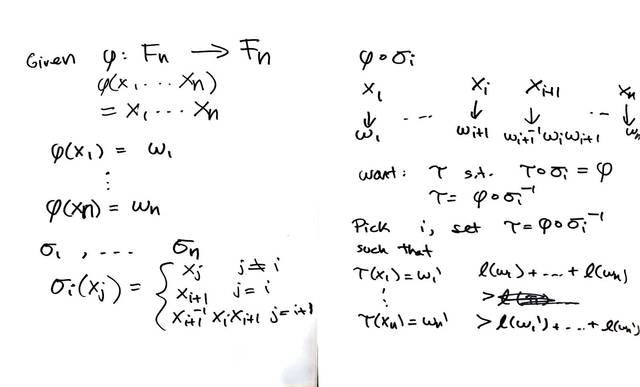200515-165501: Proving Artin's theorem.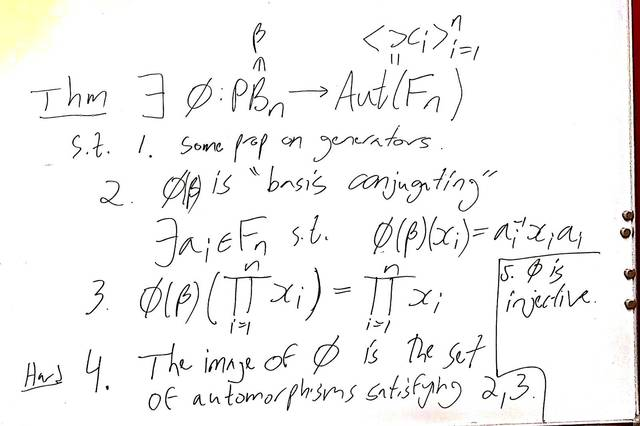200505-163633: The Artin representation.